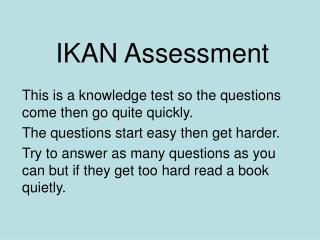DownloadDownload PresentationIKAN Assessment

# IKAN Assessment

Download Presentation## IKAN Assessment

- - - - - - - - - - - - - - - - - - - - - - - - - - - E N D - - - - - - - - - - - - - - - - - - - - - - - - - - -
##### Presentation Transcript

1. IKAN Assessment This is a knowledge test so the questions come then go quite quickly. The questions start easy then get harder. Try to answer as many questions as you can but if they get too hard read a book quietly.

2. Bank One

3. Question 1 What number is one more than 89?

4. Question 2 What number is one less than 60?

5. Question 3 Write the fraction for one quarter.

6. Question 4 Write the fraction for one third.

7. Question 5 How many tens are in 70?

8. Question 6 What is the number for six groups of ten?

9. Question 7 8 + 8 = ?

10. Question 8 Half of 14 is ..?

11. Bank Two

12. Question 1 What number is one more than 899?

13. Question 2 What number is one less than 700?

14. 1 4 1 3 Question 3 Which fraction is bigger, or ?

15. 1 2 1 6 1 5 Question 4 Write these fractions in order of size, smallest to biggest.

16. Question 5 How many tens are in 930?

17. Question 6 What is the number for 56 groups of ten?

18. Question 7 6 + 8 = ?

19. Question 8 8 x 5 = ?

20. Bank Three

21. Question 1 What number is one more than 649 999?

22. Question 2 What number is one less than 303 000?

23. 5 15 15 5 Question 3 Which number is the same as ? 20 10 3

24. Write 5 as a fraction. 2 3 Question 4

25. Question 5 How many hundreds are in 43 800?

26. Question 6 How many tenths are in all of the number, 6.2?

27. Question 7 16 - 9 = ?

28. Question 8 9 x 8 = ?

29. Bank Four

30. Which number is the same as ? 1 2 3 3 2 1 2 6 9 66 100 Question 1

31. 3 4 6 8 75 100 9 16 30 40 Question 2 Which fraction does not equal ?

32. Question 3 Which decimal is bigger, 0.307 or 0.41?

33. Question 4 Which decimal is smaller, 6.2 or 6.17?

34. Question 5 How many hundredths are in all of 7.05?

35. Question 6 54 ÷ 6 = ?

36. Question 7 • ÷ 8 = 9? What number divided by eight gives nine?

37. Question 8 Write all the factors of 32.

38. Bank Five

39. 2 3 3 5 Question 1 Which fraction is bigger, or ?

40. 2 5 3 8 Question 2 Which fraction is smaller or ?

41. Question 3 How many hundredths are in all of 2.081?

42. Question 4 What number is half-way between 7.3 and 7.4?

43. Question 5 What is the simplest fraction for 60%?

44. Question 6 What is 0.85 written as a percentage?

45. Question 7 What is the least common multiple of 4 and 6?

46. Question 8 What is the highest common factor of 24 and 32?

47. You have finished the IKAN assessment. Change your answer sheet with a partner so you can mark their answers. The next slides show the answers one by one.

48. Bank One